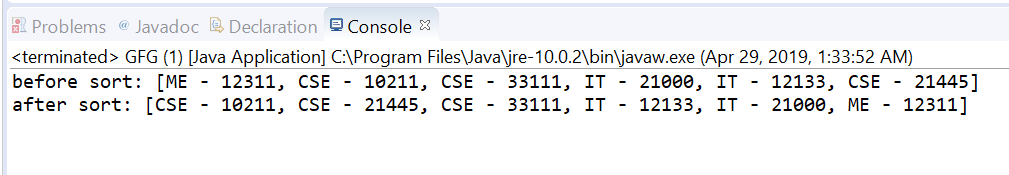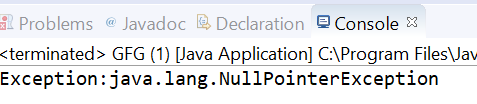# Comparator thenComparingLong() method in Java with examples

The thenComparingLong(java.util.function.ToLongFunction) method of Comparator Interface in Java returns a lexicographic-order comparator with a function that extracts a Long sort key.

Syntax:

```default Comparator <T> thenComparingLong(
ToLongFunction <T> keyExtractor)
```

Parameters: This method accepts keyExtractor which is the function used to extract the Long sort key.

Return value: This method returns a lexicographic-order comparator composed of this and then the Long sort key.

Exception: This method throws NullPointerException if the argument is null.

Below programs illustrate thenComparingLong(java.util.function.ToLongFunction) method:
Program 1:

 `// Java program to demonstrate Comparator ` `// thenComparingLong(ToLongFunction) method ` ` `  `import` `java.util.Arrays; ` `import` `java.util.Collections; ` `import` `java.util.Comparator; ` `import` `java.util.List; ` ` `  `public` `class` `GFG { ` `    ``public` `static` `void` `main(String... args) ` `    ``{ ` `        ``List list = createUsers(); ` `        ``System.out.printf(``"before sort: %s%n"``, list); ` `        ``Collections.sort(list, ` `                         ``Comparator ` `                             ``.comparing(Users::getDepartment) ` `                             ``.thenComparingLong(Users::getId)); ` `        ``System.out.printf(``"after sort: %s%n"``, list); ` `    ``} ` ` `  `    ``private` `static` `List createUsers() ` `    ``{ ` `        ``return` `Arrays.asList( ` `            ``new` `Users(``12311``, ``"ME"``), ` `            ``new` `Users(``10211``, ``"CSE"``), ` `            ``new` `Users(``33111``, ``"CSE"``), ` `            ``new` `Users(``21000``, ``"IT"``), ` `            ``new` `Users(``12133``, ``"IT"``), ` `            ``new` `Users(``21445``, ``"CSE"``)); ` `    ``} ` ` `  `    ``private` `static` `class` `Users { ` `        ``private` `long` `id; ` `        ``private` `String department; ` ` `  `        ``public` `Users(``long` `id, String department) ` `        ``{ ` `            ``this``.id = id; ` `            ``this``.department = department; ` `        ``} ` ` `  `        ``public` `long` `getId() ` `        ``{ ` `            ``return` `id; ` `        ``} ` ` `  `        ``public` `void` `setId(``long` `id) ` `        ``{ ` `            ``this``.id = id; ` `        ``} ` ` `  `        ``public` `String getDepartment() ` `        ``{ ` `            ``return` `department; ` `        ``} ` ` `  `        ``public` `void` `setDepartment(String department) ` `        ``{ ` `            ``this``.department = department; ` `        ``} ` ` `  `        ``@Override` `        ``public` `String toString() ` `        ``{ ` `            ``return` `department + ``" - "` `+ id; ` `        ``} ` `    ``} ` `} `

The output printed on console of IDE is shown below.
Output:You can see in example first sorting is done on department wise and if the department is same then ID wise.

Program 2:

 `// Java program to demonstrate Comparator ` `// thenComparingLong(ToLongFunction) method ` ` `  `import` `java.util.Arrays; ` `import` `java.util.Comparator; ` `import` `java.util.List; ` ` `  `public` `class` `GFG { ` `    ``public` `static` `void` `main(String... args) ` `    ``{ ` ` `  `        ``List list ` `            ``= Arrays.asList(``"KKR"``, ``"CSK"``, ` `                            ``"MI"``, ``"KXIP"``, ``"RCB"``, ` `                            ``"SRH"``, ``"DC"``, ``"RR"``); ` ` `  `        ``try` `{ ` ` `  `            ``// apply thenComparingLong ` `            ``Comparator.comparing(list::get) ` `                ``.thenComparingLong(``null``); ` `        ``} ` `        ``catch` `(Exception e) { ` ` `  `            ``System.out.printf(``"Exception:"` `+ e); ` `        ``} ` `    ``} ` `} `

The output printed on console is shown below.
Output: